# RD Sharma Solutions for Class 8 Math Chapter 6 - Algebraic Expressions and Identities (Part-4 ) Notes | Study RD Sharma Solutions for Class 8 Mathematics - Class 8

## Class 8: RD Sharma Solutions for Class 8 Math Chapter 6 - Algebraic Expressions and Identities (Part-4 ) Notes | Study RD Sharma Solutions for Class 8 Mathematics - Class 8

The document RD Sharma Solutions for Class 8 Math Chapter 6 - Algebraic Expressions and Identities (Part-4 ) Notes | Study RD Sharma Solutions for Class 8 Mathematics - Class 8 is a part of the Class 8 Course RD Sharma Solutions for Class 8 Mathematics.
All you need of Class 8 at this link: Class 8

#### Question 6: Multiply: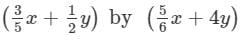#### Answer 6: To multiply, we will use distributive law as follows: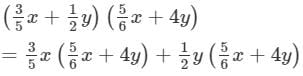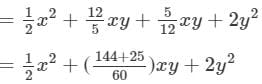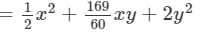Thus, the answer is#### Answer 7: To multiply, we will use distributive law as follows:

(x6y6)(x2+y2)=x6(x2+y2)y6(x2+y2)=(x8+x6y2)(y6x2+y8)=x8+x6y2y6x2y8 Thus, the answer is x8+x6y2y6x2y8x8+x6y2-y6x2-y8.

#### Answer 8: To multiply, we will use distributive law as follows:

(x2+y2)(3a+2b)=x2(3a+2b)+y2(3a+2b)=3ax2+2bx2+3ay2+2by2 Thus, the answer is 3ax2+2bx2+3ay2+2by23ax2+2bx2+3ay2+2by2.

#### Question 12: Multiply: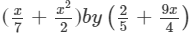#### Answer 12: To multiply the expressions, we will use the distributive law in the following way: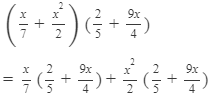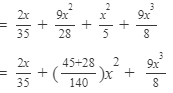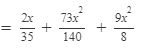Thus, the answer is#### Question 13: Multiply: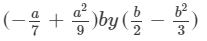#### Answer 13: To multiply, we will use distributive law as follows: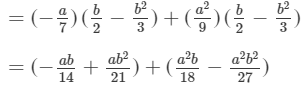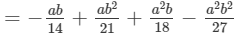Thus, the answer is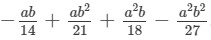#### Question 14: Multiply:

(3x2y − 5xy2) by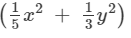#### Answer 14: To multiply, we will use distributive law as follows:

(3x2y5xy2) (1/5 x2+1/3 y2)

1/5 x2(3x2y5xy2)+1/3 y2(3x2y5xy2)

= 3/5 x4yx3y2+x2y35/3 xy4

Thus, the answer is 3/5 x4yx3y2+x2y35/3 xy4

#### Answer 18: To multiply, we will  use distributive law as follows:

(x2y1)(32x2y)=x2y(32x2y)1×(32x2y)=3x2y2x4y23+2x2y=5x2y2x4y23  (x2y1)(32x2y)=5x2y2x4y23x2y-13-2x2y=5x2y-2x4y2-3

Now, we put x = -1 and y = -2 on both sides to verify the result.

LHS = (x2y1)(32x2y)=[(1)2(2)1][32(1)2(2)]=[1×(2)1][32×1×(2)]=(21)(3+4)=3×7=21RHS=5x2y2x4y23=5(1)2(2)2(1)4(2)23=[5×1×(2)][2×1×4]3=1083=21  Because LHS is equal to RHS, the result is verified.

#### Question 19: Find the following product and verify the result for x = − 1, y = − 2:#### Answer 19: To multiply, we will use distributive law as follows: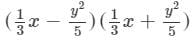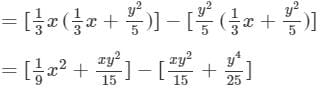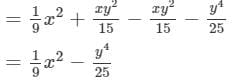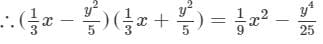Now, we will put x = -1 and y = -2 on both the sides to verify the result.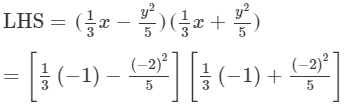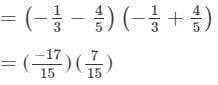=- 119/225

RHS=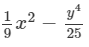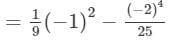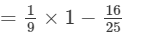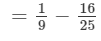= - 119/225

Because LHS is equal to RHS, the result is verified.

Thus, the answer is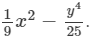#### Answer 20: To simplify, we will proceed as follows:

x2(x+2y)(x3y)=[x2(x+2y)](x3y)=(x3+2x2y)(x3y)=x3(x3y)+2x2y(x3y)=x43x3y+2x3y6x2y2=x4x3y6x2y2 Thus, the answer is x4x3y6x2y2x4-x3y-6x2y2.

#### Answer 21: To simplify, we will proceed as follows:

(x22y2)(x+4y)x2y2=[x2(x+4y)2y2(x+4y)]x2y2=(x3+4x2y2xy28y3)x2y2=x5y2+4x4y32x3y48x2y5 Thus, the answer is x5y2+4x4y32x3y48x2y5x5y2+4x4y3-2x3y4-8x2y5.

#### Answer 22: To simplify, we will proceed as follows:

a2b2(a+2b)(3a+b)=[a2b2(a+2b)](3a+b)=(a3b2+2a2b3)(3a+b)=3a(a3b2+2a2b3)+b(a3b2+2a2b3)=3a4b2+6a3b3+a